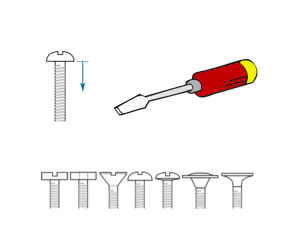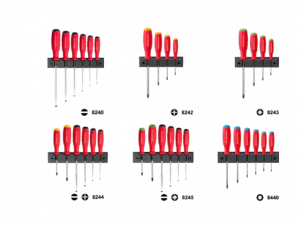## Go 编程模式：Go Generation图片来源：GopherSource

### 本文是全系列中第6 / 9篇：Go编程模式

«上一篇文章下一篇文章»

#### 现实中的类比#### Go语方的类型检查

Type Assert

```//Container is a generic container, accepting anything.
type Container []interface{}

//Put adds an element to the container.
func (c *Container) Put(elem interface{}) {
*c = append(*c, elem)
}
//Get gets an element from the container.
func (c *Container) Get() interface{} {
elem := (*c)
*c = (*c)[1:]
return elem
}```

```intContainer := &Container{}
intContainer.Put(7)
intContainer.Put(42)```

```// assert that the actual type is int
elem, ok := intContainer.Get().(int)
if !ok {
fmt.Println("Unable to read an int from intContainer")
}

fmt.Printf("assertExample: %d (%T)\n", elem, elem)
```
Reflection

```type Container struct {
s reflect.Value
}
func NewContainer(t reflect.Type, size int) *Container {
if size <=0  { size=64 }
return &Container{
s: reflect.MakeSlice(reflect.SliceOf(t), 0, size),
}
}
func (c *Container) Put(val interface{})  error {
if reflect.ValueOf(val).Type() != c.s.Type().Elem() {
return fmt.Errorf(“Put: cannot put a %T into a slice of %s",
val, c.s.Type().Elem()))
}
c.s = reflect.Append(c.s, reflect.ValueOf(val))
return nil
}
func (c *Container) Get(refval interface{}) error {
if reflect.ValueOf(refval).Kind() != reflect.Ptr ||
reflect.ValueOf(refval).Elem().Type() != c.s.Type().Elem() {
return fmt.Errorf("Get: needs *%s but got %T", c.s.Type().Elem(), refval)
}
reflect.ValueOf(refval).Elem().Set( c.s.Index(0) )
c.s = c.s.Slice(1, c.s.Len())
return nil
}```

• 在NewContainer()会根据参数的类型初始化一个Slice
• 在Put()时候，会检查val是否和Slice的类型一致。
• 在Get()时，我们需要用一个入参的方式，因为我们没有办法返回reflect.Value或是interface{}，不然还要做Type Assert
• 但是有类型检查，所以，必然会有检查不对的道理 ，因此，需要返回error

```f1 := 3.1415926
f2 := 1.41421356237

c := NewMyContainer(reflect.TypeOf(f1), 16)

if err := c.Put(f1); err != nil {
panic(err)
}
if err := c.Put(f2); err != nil {
panic(err)
}

g := 0.0

if err := c.Get(&g); err != nil {
panic(err)
}
fmt.Printf("%v (%T)\n", g, g) //3.1415926 (float64)
fmt.Println(c.s.Index(0)) //1.4142135623```

#### 它山之石

 ```//用来描述泛型 template T GetMax (T a, T b) { T result; result = (a>b)? a : b; return (result); } ``` ```int i=5, j=6, k; //生成int类型的函数 k=GetMax(i,j); long l=10, m=5, n; //生成long类型的函数 n=GetMax(l,m); ```

C++的编译器会在编译时分析代码，根据不同的变量类型来自动化的生成相关类型的函数或类。C++叫模板的具体化。

#### Go Generator

1. 一个函数模板，其中设置好相应的占位符。
2. 一个脚本，用于按规则来替换文本并生成新的代码。
3. 一行注释代码。

```package PACKAGE_NAME
type GENERIC_NAMEContainer struct {
s []GENERIC_TYPE
}
func NewGENERIC_NAMEContainer() *GENERIC_NAMEContainer {
return &GENERIC_NAMEContainer{s: []GENERIC_TYPE{}}
}
func (c *GENERIC_NAMEContainer) Put(val GENERIC_TYPE) {
c.s = append(c.s, val)
}
func (c *GENERIC_NAMEContainer) Get() GENERIC_TYPE {
r := c.s
c.s = c.s[1:]
return r
}```

• PACKAGE_NAME– 包名
• GENERIC_NAME– 名字
• GENERIC_TYPE– 实际的类型

```#!/bin/bash

set -e

SRC_FILE=\${1}
PACKAGE=\${2}
TYPE=\${3}
DES=\${4}
#uppcase the first char
PREFIX="\$(tr '[:lower:]' '[:upper:]' <<< \${TYPE:0:1})\${TYPE:1}"

DES_FILE=\$(echo \${TYPE}| tr '[:upper:]' '[:lower:]')_\${DES}.go

sed 's/PACKAGE_NAME/'"\${PACKAGE}"'/g' \${SRC_FILE} | \
sed 's/GENERIC_TYPE/'"\${TYPE}"'/g' | \
sed 's/GENERIC_NAME/'"\${PREFIX}"'/g' > \${DES_FILE}```

• 模板源文件
• 包名
• 实际需要具体化的类型
• 用于构造目标文件名的后缀

```//go:generate ./gen.sh ./template/container.tmp.go gen uint32 container
func generateUint32Example() {
var u uint32 = 42
c := NewUint32Container()
c.Put(u)
v := c.Get()
fmt.Printf("generateExample: %d (%T)\n", v, v)
}

//go:generate ./gen.sh ./template/container.tmp.go gen string container
func generateStringExample() {
var s string = "Hello"
c := NewStringContainer()
c.Put(s)
v := c.Get()
fmt.Printf("generateExample: %s (%T)\n", v, v)
}```

• 第一个注释是生成包名为gen类型为uint32目标文件名以container 为后缀
• 第二个注释是生成包名为gen类型为string目标文件名以container为后缀

```package gen

type Uint32Container struct {
s []uint32
}
func NewUint32Container() *Uint32Container {
return &Uint32Container{s: []uint32{}}
}
func (c *Uint32Container) Put(val uint32) {
c.s = append(c.s, val)
}
func (c *Uint32Container) Get() uint32 {
r := c.s
c.s = c.s[1:]
return r
}```

```package gen

type StringContainer struct {
s []string
}
func NewStringContainer() *StringContainer {
return &StringContainer{s: []string{}}
}
func (c *StringContainer) Put(val string) {
c.s = append(c.s, val)
}
func (c *StringContainer) Get() string {
r := c.s
c.s = c.s[1:]
return r
}
```

#### 新版Filter

```package PACKAGE_NAME

type GENERIC_NAMEList []GENERIC_TYPE

type GENERIC_NAMEToBool func(*GENERIC_TYPE) bool

func (al GENERIC_NAMEList) Filter(f GENERIC_NAMEToBool) GENERIC_NAMEList {
var ret GENERIC_NAMEList
for _, a := range al {
if f(&a) {
ret = append(ret, a)
}
}
return ret
}
```

```type Employee struct {
Name     string
Age      int
Vacation int
Salary   int
}

//go:generate ./gen.sh ./template/filter.tmp.go gen Employee filter
func filterEmployeeExample() {

var list = EmployeeList{
{"Hao", 44, 0, 8000},
{"Bob", 34, 10, 5000},
{"Alice", 23, 5, 9000},
{"Jack", 26, 0, 4000},
{"Tom", 48, 9, 7500},
}

var filter EmployeeList
filter = list.Filter(func(e *Employee) bool {
return e.Age > 40
})

fmt.Println("----- Employee.Age > 40 ------")
for _, e := range filter {
fmt.Println(e)
}

filter = list.Filter(func(e *Employee) bool {
return e.Salary <= 5000
})

fmt.Println("----- Employee.Salary <= 5000 ------")
for _, e := range filter {
fmt.Println(e)
}
}```

#### 第三方工具

（全文完）（转载本站文章请注明作者和出处酷 壳 – CoolShell，请勿用于任何商业用途）

——===访问酷壳404页面寻找遗失儿童。===——
The postGo 编程模式：Go Generationfirst appeared on酷 壳 - CoolShell.

[返回] [原文链接]